2023 Vezina Trophy Winner Odds
+400
4 to 1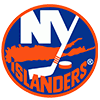Ilya Sorokin
13.6% implied probability

+600
6 to 1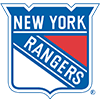Igor Shesterkin
9.7% implied probability

+600
6 to 1Juuse Saros
9.7% implied probability

+700
7 to 1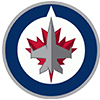Connor Hellebuyck
8.5% implied probability

+800
8 to 1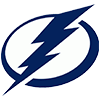Andrei Vasilevskiy
7.5% implied probability

+1200
12 to 1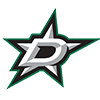Jake Oettinger
5.2% implied probability

+1800
18 to 1Linus Ullmark
3.6% implied probability

+2000
20 to 1Filip Gustavsson
3.2% implied probability

+2500
25 to 1Alexandar Georgiev
2.6% implied probability

+3000
30 to 1Thatcher Demko
2.2% implied probability

+3000
30 to 1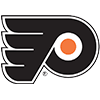Carter Hart
2.2% implied probability

+3000
30 to 1Jeremy Swayman
2.2% implied probability

+3000
30 to 1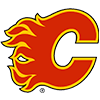Jacob Markstrom
2.2% implied probability

+4000
40 to 1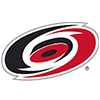Frederik Andersen
1.7% implied probability

+4000
40 to 1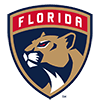Sergei Bobrovsky
1.7% implied probability

+4000
40 to 1Pyotr Kochetkov
1.7% implied probability

+5000
50 to 1Devon Levi
1.3% implied probability

+5000
50 to 1Tristan Jarry
1.3% implied probability

+5000
50 to 1John Gibson
1.3% implied probability

+5000
50 to 1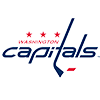Darcy Kuemper
1.3% implied probability

+5000
50 to 1Logan Thompson
1.3% implied probability

+5000
50 to 1Karel Vejmelka
1.3% implied probability

+5000
50 to 1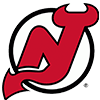Akira Schmid
1.3% implied probability

+7500
75 to 1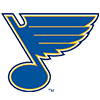Jordan Binnington
0.9% implied probability

+7500
75 to 1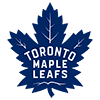Ilya Samsonov
0.9% implied probability

+7500
75 to 1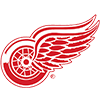Ville Husso
0.9% implied probability

+8000
80 to 1Adin Hill
0.8% implied probability

+10000
100 to 1Jesper Wallstedt
0.7% implied probability

+10000
100 to 1Dustin Wolf
0.7% implied probability

+10000
100 to 1Joseph Woll
0.7% implied probability

+10000
100 to 1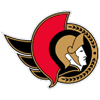Mads Sogaard
0.7% implied probability

+10000
100 to 1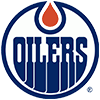Stuart Skinner
0.7% implied probability

+10000
100 to 1Marc-Andre Fleury
0.7% implied probability

+10000
100 to 1Jack Campbell
0.7% implied probability

+10000
100 to 1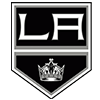Cam Talbot
0.7% implied probability

+10000
100 to 1Semyon Varlamov
0.7% implied probability

+15000
150 to 1Dan Vladar
0.4% implied probability

+15000
150 to 1Vitek Vanecek
0.4% implied probability

+15000
150 to 1Antti Raanta
0.4% implied probability

+15000
150 to 1Sebastian Cossa
0.4% implied probability

+15000
150 to 1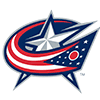Elvis Merzlikins
0.4% implied probability

+30000
300 to 1Jonathan Quick
0.2% implied probability

+30000
300 to 1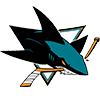Kaapo Kahkonen
0.2% implied probability

+30000
300 to 1Alex Nedeljkovic
0.2% implied probability

+30000
300 to 1Eric Comrie
0.2% implied probability

+30000
300 to 1Anton Forsberg
0.2% implied probability

+30000
300 to 1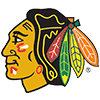Petr Mrazek
0.2% implied probability

+30000
300 to 1James Reimer
0.2% implied probability